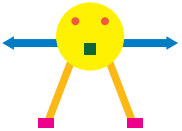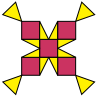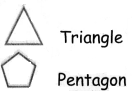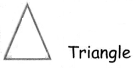Students can download 4th Maths Term 1 Chapter 1 Geometry Ex 1.1 Questions and Answers, Notes, Samacheer Kalvi 4th Maths Guide Pdf helps you to revise the complete Tamilnadu State Board New Syllabus, helps students complete homework assignments and to score high marks in board exams.

## Tamilnadu Samacheer Kalvi 4th Maths Solutions Term 1 Chapter 1 Geometry Ex 1.1

A. Write down the names of shape in the following pictures.

(i)Circle, square, rectangle, triangle(ii)Square, circle, triangle, rectangle

B.
(i) Write the number of squares and triangles in the given picture.Square _______
Triangle _______
Square     5
Triangle    12(ii) Write the number of rectangles and triangles in the given pictureRectangle _______
Triangle ______